Next: Electromagnetic radiation Up: Electromagnetic energy and momentum Previous: Electromagnetic momentum

## Momentum conservation

It follows from Eqs. (1036) and (1050) that the momentum density of electromagnetic fields can be written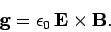(1058)

Now, a momentum conservation equation for electromagnetic fields should take the integral form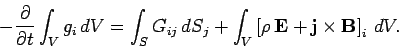(1059)

Here,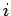and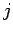run from 1 to 3 (1 corresponds to the-direction, 2 to the-direction, and 3 to the-direction). Moreover, the Einstein summation convention is employed for repeated indices (e.g.,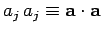). Furthermore, the tensor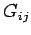represents the flux of theth component of electromagnetic momentum in the-direction. This tensor (a tensor is a direct generalization of a vector with two indices instead of one) is called the momentum flux density. Hence, the above equation states that the rate of loss of electromagnetic momentum in some volumeis equal to the flux of electromagnetic momentum across the bounding surfaceplus the rate at which momentum is transferred to matter inside. The latter rate is, of course, just the net electromagnetic force acting on matter inside: i.e., the volume integral of the electromagnetic force density,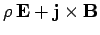. Now, a direct generalization of the divergence theorem states that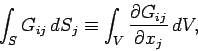(1060)

where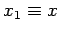,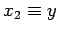, etc. Hence, in differential form, our momentum conservation equation for electromagnetic fields is written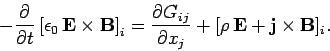(1061)

Let us now attempt to derive an equation of this form from Maxwell's equations.

Maxwell's equations are written: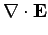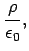(1062)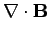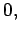(1063)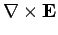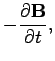(1064)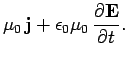(1065)

We can cross Eq. (1065) divided by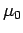with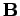, and rearrange, to give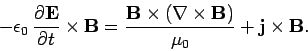(1066)

Next, let us cross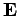with Eq. (1064) times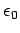, rearrange, and add the result to the above equation. We obtain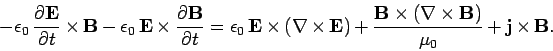(1067)

Next, making use of Eqs. (1062) and (1063), we get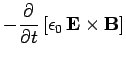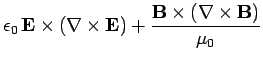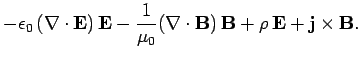(1068)

Now, from vector field theory,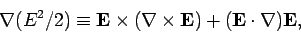(1069)

with a similar equation for. Hence, Eq. (1068) takes the form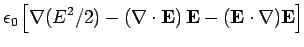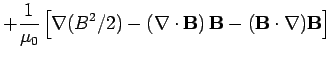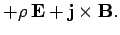(1070)

Finally, when written in terms of components, the above equation becomes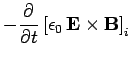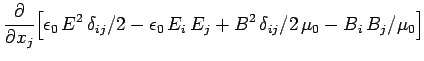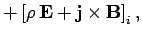(1071)

since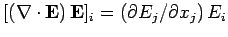, and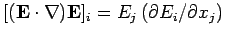. Here,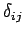is a Kronecker delta symbol (i.e.,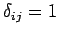if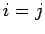, and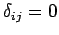otherwise). Comparing the above equation with Eq. (1061), we conclude that the momentum flux density tensor of electromagnetic fields takes the form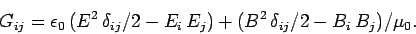(1072)

The momentum conservation equation (1061) is sometimes written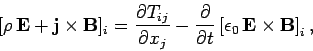(1073)

where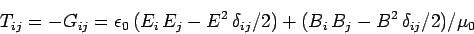(1074)

is called the Maxwell stress tensor.Next: Electromagnetic radiation Up: Electromagnetic energy and momentum Previous: Electromagnetic momentum
Richard Fitzpatrick 2006-02-02